Home > GC > Chapter 6 > Lesson 6.2.2 > Problem6-54

6-54.

the diagram at right. Find two equivalent expressions that represent the area of the inner square. Homework Help ✎

Multiple Choice: To decide if his class would take a quiz today, he will flip a coin three times. If all three results are heads or all three results are tails, he will give the quiz. Otherwise, his students will not be tested. What is the probability that his class will take the quiz? Homework Help ✎

1.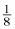2.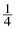3.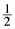4. 1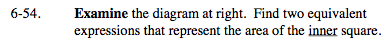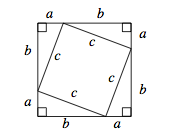Area of inner square = (base)(height) or large square minus 4 triangles.

c² or a² + b²